# Gon functions

Decide which of the numbers (values ​​of trigonometric functions) are positive and which are negative (or zero). Positive mark +1 and negative -1.

Correct result:

sin (-33°):  -1
sin (889°):  1
sin (-432°):  -1
cos (919°):  -1
cos (-16°):  1
cos (-38°):  1
cos (222°):  -1
cos (546°):  -1
sin (-403°):  -1
sin (-9°):  -1

#### Solution:

sin (-33°) = -0.5446 < 0; -33 ° ... Q4; sin Q4 < 0
sin (889°) = 0.1908 > 0; 889 ° ... Q2; sin Q2 > 0
sin (-432°) = -0.9511 < 0; -432 ° ... Q4; sin Q4 < 0
cos (919°) = -0.9455 < 0; 919 ° ... Q3; cos Q3 < 0
cos (-16°) = 0.9613 > 0; -16 ° ... Q4; cos Q4 > 0
cos (-38°) = 0.788 > 0; -38 ° ... Q4; cos Q4 > 0
cos (222°) = -0.7431 < 0; 222 ° ... Q3; cos Q3 < 0
cos (546°) = -0.9945 < 0; 546 ° ... Q3; cos Q3 < 0
sin (-403°) = -0.682 < 0; -403 ° ... Q4; sin Q4 < 0
sin (-9°) = -0.1564 < 0; -9 ° ... Q4; sin Q4 < 0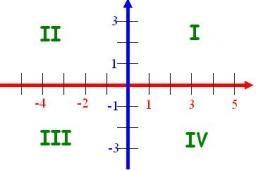We would be very happy if you find an error in the example, spelling mistakes, or inaccuracies, and please send it to us. We thank you!Tips to related online calculators

#### You need to know the following knowledge to solve this word math problem:

We encourage you to watch this tutorial video on this math problem:

## Next similar math problems:

• ClimbFor horizontal distance 4.2 km road rise by 6.3 m. Calculate the road pitch in ‰ (permille, parts per thousand).
• Obtuse angle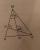The line OH is the height of the triangle DOM, line MN is the bisector of angle DMO. obtuse angle between the lines MN and OH is four times larger than the angle DMN. What size is the angle DMO? (see attached image)
• The dormitoryThe dormitory accommodates 150 pupils in 42 rooms, some of which are triple and some are quadruple. Determine how many rooms are triple and how many quadruples.
• Two numbersWe have two numbers. Their sum is 140. One-fifth of the first number is equal to half the second number. Determine those unknown numbers.
• Calculate 2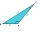Calculate the largest angle of the triangle whose side are 5.2cm, 3.6cm, and 2.1cm
• CosineThe point (8, 6) is on the terminal side of angle θ. cos θ = ?
• FlowerbedFlowerbed has the shape of an isosceles obtuse triangle. Arm has a size 5.5 meters and an angle opposite to the base size is 94°. What is the distance from the base to opposite vertex?
• Center traverseIt is true that the middle traverse bisects the triangle?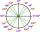What is greater angle? -0.7 radians or -0.7π radians?
• Find the 9Find the missing angle in the triangle and then name triangle. Angles are: 95, 2x+15, x+3
• Similarity coefficient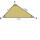The triangles ABC and A "B" C "are similar to the similarity coefficient 2. The sizes of the angles of the triangle ABC are α = 35° and β = 48°. Find the magnitudes of all angles of triangle A "B" C ".
• SlopeFind the slope of the line: x=t and y=1+t.
• Clock handsCalculate the internal angles of a triangle whose vertices lie on the clock's 2, 6 and 11 hours.
• Three brothersThe three brothers have a total of 42 years. Jan is five years younger than Peter and Peter is 2 years younger than Michael. How many years has each of them?
• Equations - simpleSolve system of linear equations: x-2y=6 3x+2y=4
• Triangle SSAConstruct a triangle ABC if |AB| = 5cm va = 3cm, CAB = 50 °. It is to create the analysis and construction steps.
• Ruler and compassUse a ruler and compass to construct a triangle ABC with AB 5cm BAC 60° and ACB 45°.